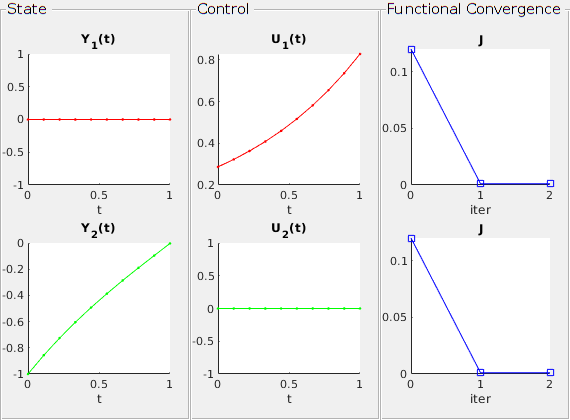# Basic example of Pontryagin Problems

To start this tutorial, write the following command in the MATLAB console

open T041_BasicExample

DyCon toolbox adopts Pontryagin’s maximum principle to optimize the control function for each control problem. In this way we can solve problems of the form:

subject to

‘Pontryagin’ class uses symbolic interface to define a control problem as in ‘ode’ class. ‘Pontryagin’ class contains ‘ode’ class, the final cost, and running cost. As ‘ode’ class contains $f$, the final cost $\Psi$ and running cost $L$ should be given by symbolic functions.

Here we explain ‘Pontryagin’ class with a simple example: we want to minimize the objective function

subject to

The dynamics and cost functions are based on symbolic vectors $Y$ and $U$, which represent the state of the dynamics and control vector.

Y = sym('y',[2 1])


Y =

y1
y2


U = sym('u',[2 1])


U =

u1
u2



The dynamics of $Y$ should be given by a symbolic vector with the same dimensions as the state vector. Following the notation at the beginning, this vector represents $f (t, Y, U)$:

mu = param('mu',10);
nu = param('nu',1);
%%
F = @(t,Y,U,Params) [      Y(1)*Y(2)  + U(2)*Params(1)        ; ...
-Y(2)       + U(1)*norm(Params(2)) ] ;


Using this dynamics vector, we construct an ‘ode’ class.

dynamics = ode(F,Y,U,[mu nu]);


The printed information above shows the default setting of this ‘ode’ class. In order to construct the dynamics we want, we need to customize its parameters. In this case we change the initial condition for the dynamics and the sampling timestep.

The time discretization is generated as a uniform mesh from the timestep $dt$. It will be used not only for the sampling of the state vector $Y$ but also to represent the control vector $U$ and cost $J$ in ‘Pontryagin’ class.

dynamics.InitialCondition = [0; -1];
dynamics.Nt = 10;


Next we need to define the functional $J$ we want to minimize. Following the form presented in , we define the expressions of $\ Psi$ and $L$ in symbolic form:

Psi = @(T,Y)   Y.'*Y;
L   = @(t,Y,U) 0.005*(U.'*U);


We finally define the optimal control problem as a ‘Pontryagin’ class:

iP = Pontryagin(dynamics,Psi,L);


This class contains information we need to find the optimal control vector $U$. It is worth mentioning that until now we defined the problem but not solved it yet.

iP


iP =

Pontryagin with properties:

Target: []
Functional: [1x1 PontryaginFunctional]
Dynamics: [1x1 ode]
Hamiltonian: [1x1 SymNumFun]
Hessian: [1x1 SymNumFun]
AnalyticalControl: [1x1 SymNumFun]
Solution: []
Constraints: [1x1 constraints]



DyCon toolbox uses the gradient methods to optimize the cost functional. This calculates the gradient of $J$ along $U$ from the first order approximation of the Hamiltonian and adjoint state vector in the Pontryagin principle.

To solve the problem using the default gradient method, we simply write:

U0 = zeros(iP.Dynamics.Nt,iP.Dynamics.ControlDimension);


Solve with precision:

We obtain: J(u) = 1.148222E-03

error = 2.000023E-03

With 3 iterations,     In 0.083441 seconds



This command generates ‘solution’ in the ‘Pontryagin’ class, which contains the optimal control vector ‘UOptimal’ and its information, such as the cost, precision and time of computations.

iP.Solution


ans =

solution with properties:

precision: 0.0020
iter: 3
time: 0.0834
Y0history: []
Yhistory: {[10x2 double]  [10x2 double]  [10x2 double]}
ControlHistory: {[10x2 double]  [10x2 double]  [10x2 double]}
Jhistory: [0.1200 0.0011 0.0011]
Phistory: {[10x2 double]  [10x2 double]  [10x2 double]}
dJhistory: {[10x2 double]  [10x2 double]  [10x2 double]}
fhistory: []
dfhistory: []
Ehistory: [0 0.0020 0.0020]
timeline: []
du: []
UOptimal: [10x2 double]
JOptimal: 0.0011



This structure is independent of the solver, and we can see the results through visualization functions we want. One of the examples is ‘plot’ function which can be applied to ‘Pontryagin’ class.

plot(iP)